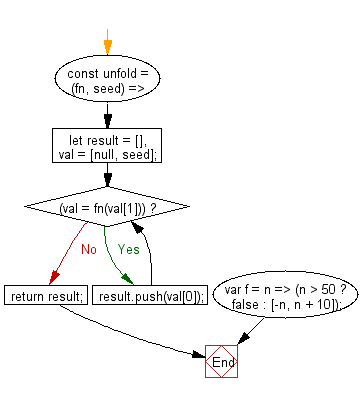# JavaScript: Build an array, using an iterator function and an initial seed value

## JavaScript fundamental (ES6 Syntax): Exercise-111 with Solution

Write a JavaScript program to build  an array, using an iterator function and an initial seed value.

• Use a while loop and Array.prototype.push() to call the function repeatedly until it returns false.
• The iterator function accepts one argument (seed) and must always return an array with two elements ([value, nextSeed]) or false to terminate.

Sample Solution:

JavaScript Code:

``````//#Source https://bit.ly/2neWfJ2
const unfold = (fn, seed) => {
let result = [],
val = [null, seed];
while ((val = fn(val))) result.push(val);
return result;
};
var f = n => (n > 50 ? false : [-n, n + 10]);
console.log(unfold(f, 10));
```
```

Sample Output:

```[-10,-20,-30,-40,-50]
```

Flowchart:Live Demo:

See the Pen javascript-basic-exercise-111-1 by w3resource (@w3resource) on CodePen.

Improve this sample solution and post your code through Disqus

What is the difficulty level of this exercise?

Test your Programming skills with w3resource's quiz.

﻿

## JavaScript: Tips of the Day

Returns an array of n-tuples of consecutive elements

Example:

```const tips_arr = (n, arr) =>  n > arr.length ? [] : arr.slice(n - 1).map((v, i) =>[...arr.slice(i, i + n - 1), v]);
console.log(tips_arr(2, [1, 2, 3, 4, 5]));
console.log(tips_arr(3, [1, 2, 3, 4, 5]));
console.log(tips_arr(5, [1, 2, 3, 4]));
```

Output:

```[[1, 2], [2, 3], [3, 4], [4, 5]]
[[1, 2, 3], [2, 3, 4], [3, 4, 5]]
[]
```